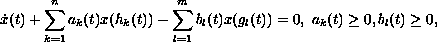Electron. J. Diff. Eqns., Vol. 2003(2003), No. 47, pp. 1-25.

### Oscillation for equations with positive and negative coefficients and distributed delay II: Applications Leonid Berezansky & Elena Braverman

Abstract:
We apply the results of our previous paper "Oscillation of equations with positive and negative coefficients and distributed delay I: General results" to the study of oscillation properties of equations with several delays and positive and negative coefficientsintegrodifferential equations with oscillating kernels and mixed equations combining two above equations. Comparison theorems, explicit non-oscillation and oscillation results are presented.

Submitted February 14, 2003. Published April 24, 2003.
Math Subject Classifications: 34K11, 34K15.
Key Words: Oscillation, non-oscillation, distributed delay, equations with several delays, integrodifferential equations.

Show me the PDF file (272K), TEX file, and other files for this article.

A related article has been published by the same authors in this journal: Oscillation for equations with positive and negative coefficients and with distributed delay I: General results, Vol. 2003(2003), No. 12, pp. 1-21.Leonid Berezansky Department of Mathematics Ben-Gurion University of the Negev Beer-Sheva 84105, Israel e-mail: brznsky@cs.bgu.ac.il Elena Braverman Department of Mathematics and Statistics University of Calgary 2500 University Drive N. W., Calgary, Alberta, Canada, T2N 1N4 Fax: (403)-282-5150, phone: (403)-220-3956 email: maelena@math.ucalgary.ca